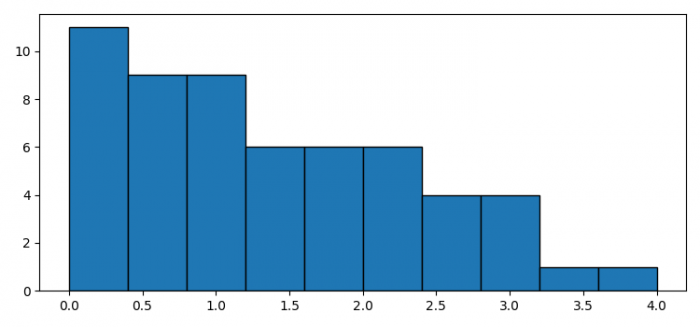# How to get a reverse-order cumulative histogram in Matplotlib?

To get a reverse-order cumulative histogram in Matplotlib, we can use cumulative = -1 in the hist() method.

• Set the figure size and adjust the padding between and around the subplots.
• Make a list of data points.
• Plot a histogram with data and cumulative = -1.
• To display the figure, use show() method.

## Example

from matplotlib import pyplot as plt

plt.rcParams["figure.figsize"] = [7.50, 3.50]
plt.rcParams["figure.autolayout"] = True

data = [1, 2, 2, 3, 1, 4, 3, 0, 1, 3, 0]

plt.hist(data, edgecolor='black', align="mid", cumulative=-1)

plt.show()

## OutputUpdated on: 07-Jul-2021

1K+ Views